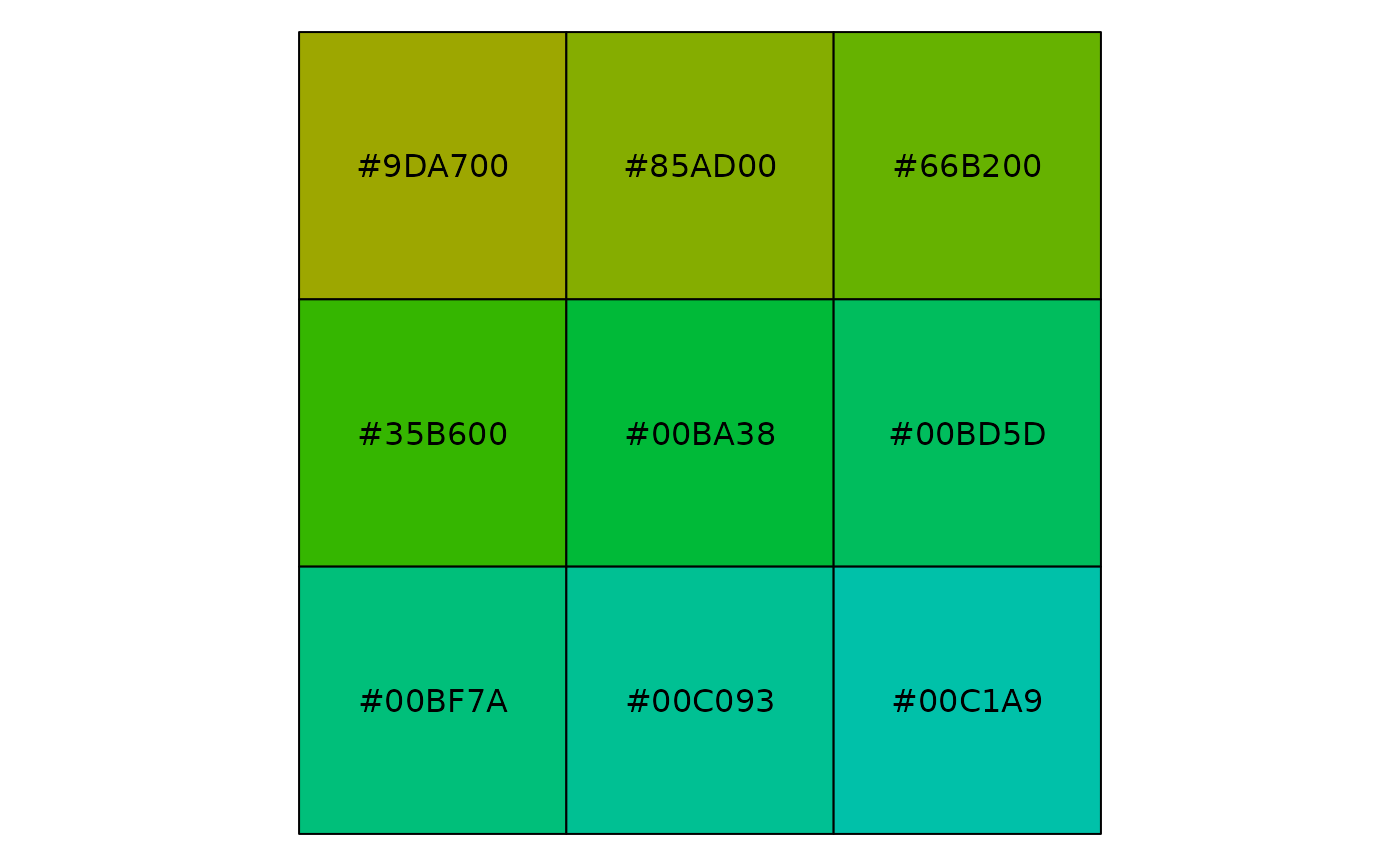Hue palette (discrete)

hue_pal(h = c(0, 360) + 15, c = 100, l = 65, h.start = 0, direction = 1)

## Arguments

h range of hues to use, in [0, 360] chroma (intensity of colour), maximum value varies depending on combination of hue and luminance. luminance (lightness), in [0, 100] hue to start at direction to travel around the colour wheel, 1 = clockwise, -1 = counter-clockwise

## Examples

show_col(hue_pal()(4))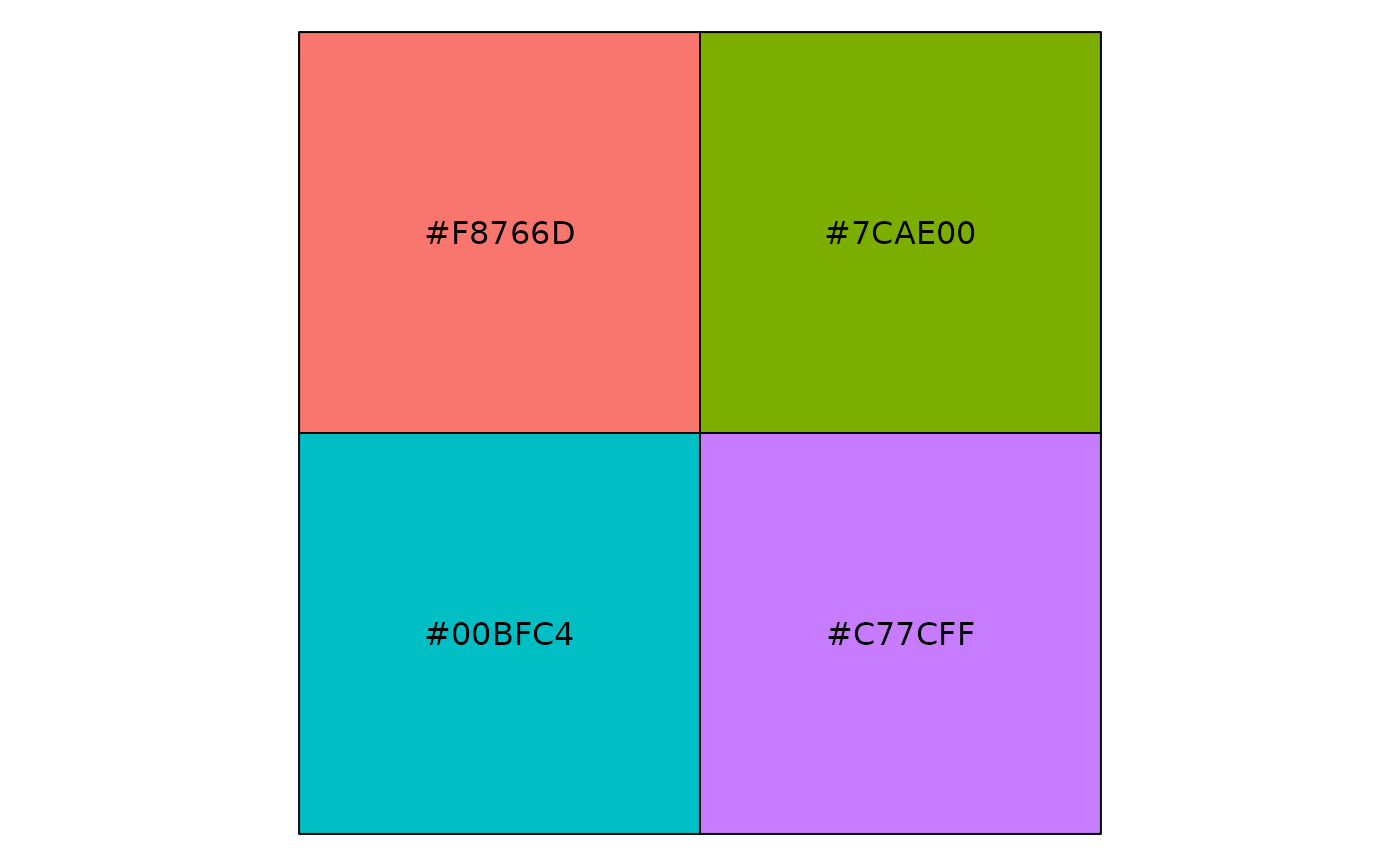show_col(hue_pal()(9))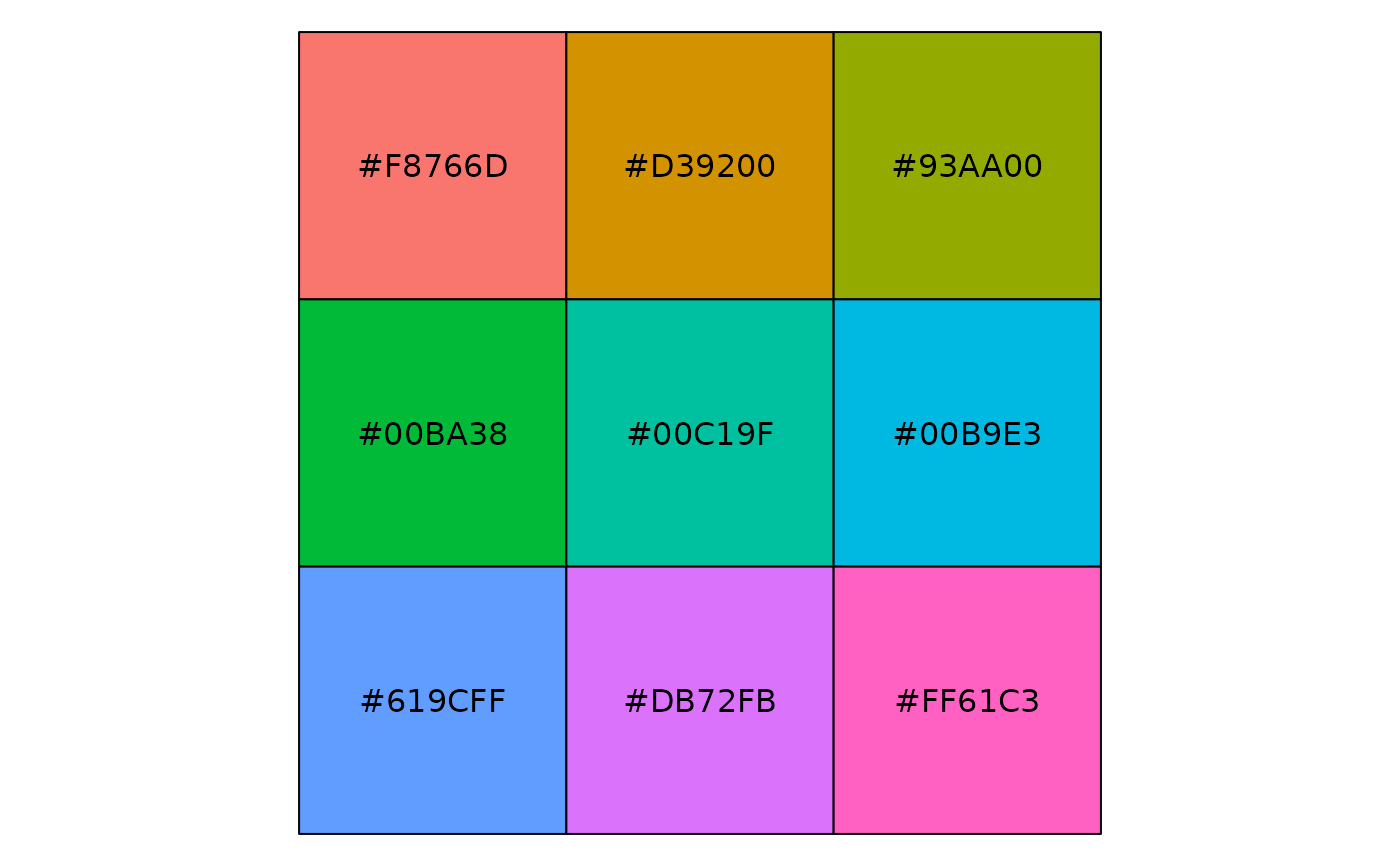show_col(hue_pal(l = 90)(9))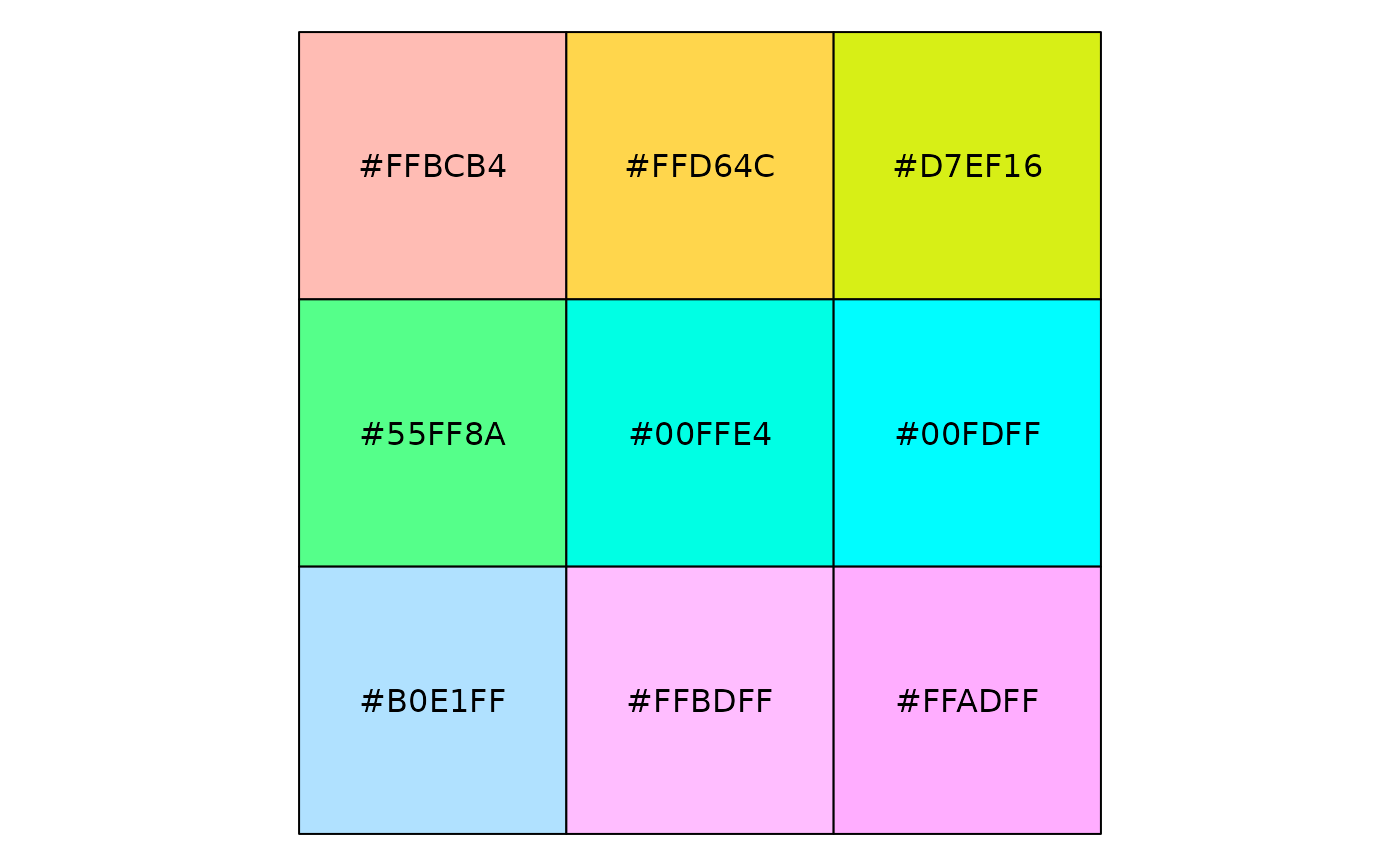show_col(hue_pal(l = 30)(9))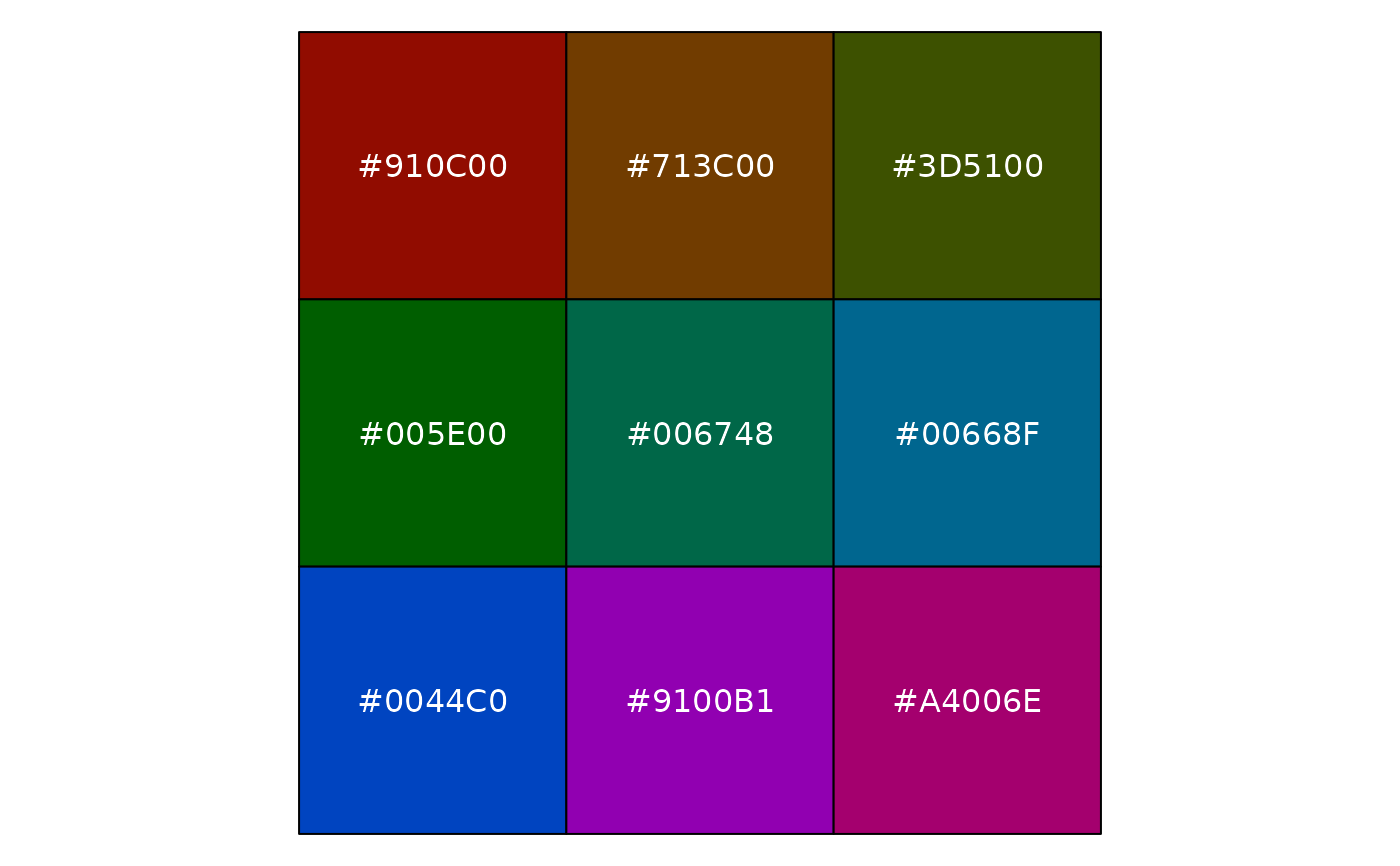show_col(hue_pal()(9))show_col(hue_pal(direction = -1)(9))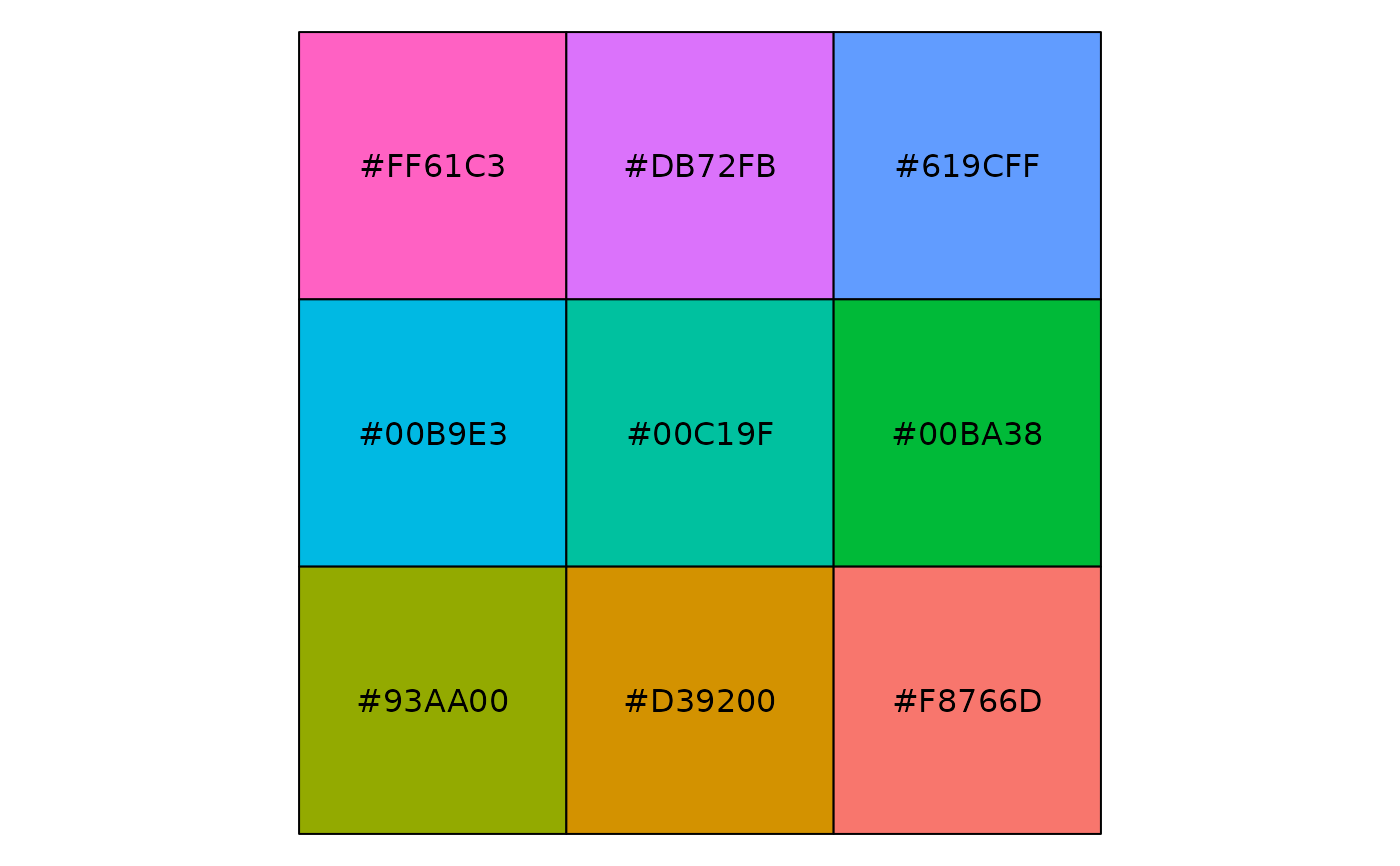show_col(hue_pal()(9))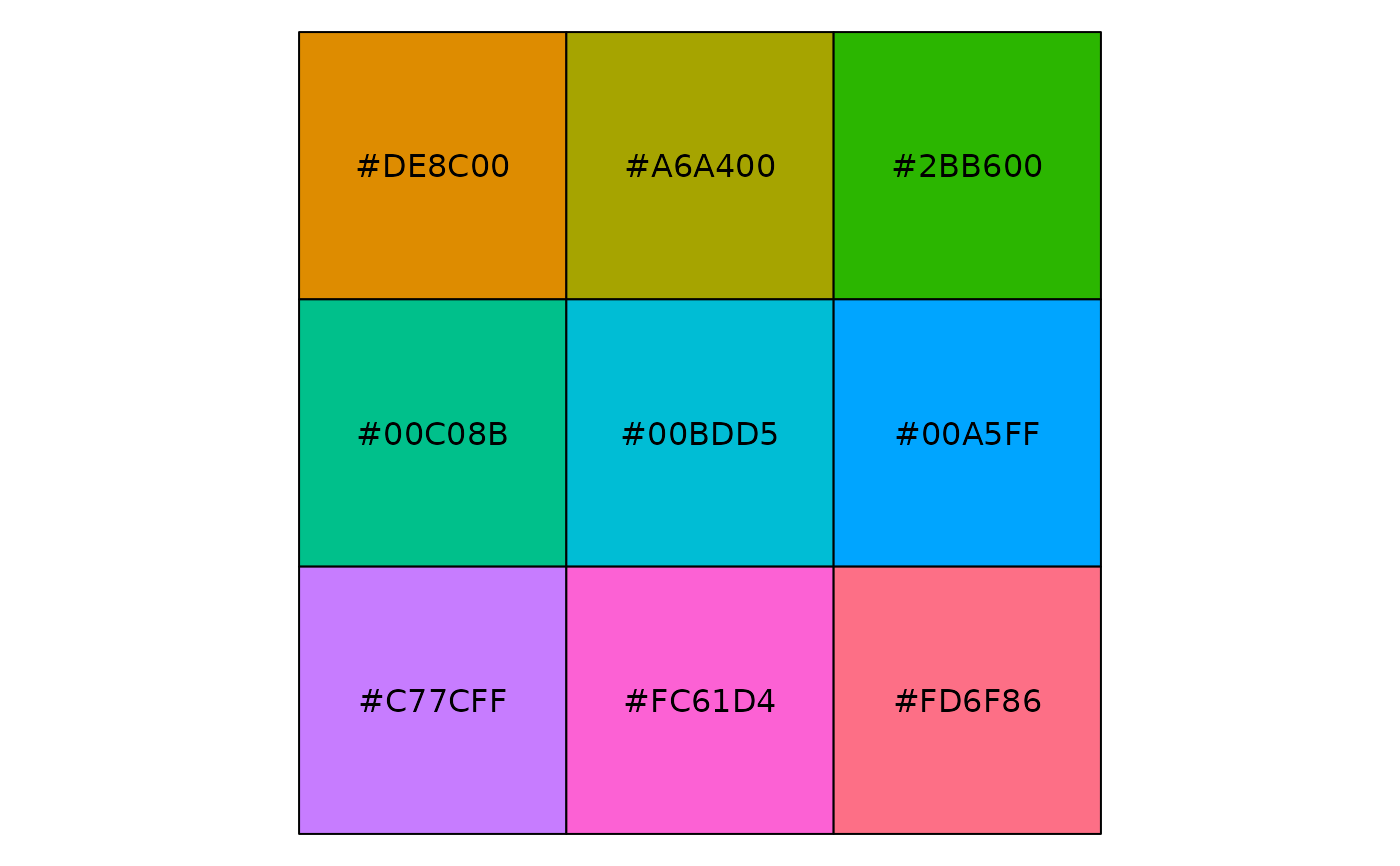show_col(hue_pal(h = c(0, 90))(9))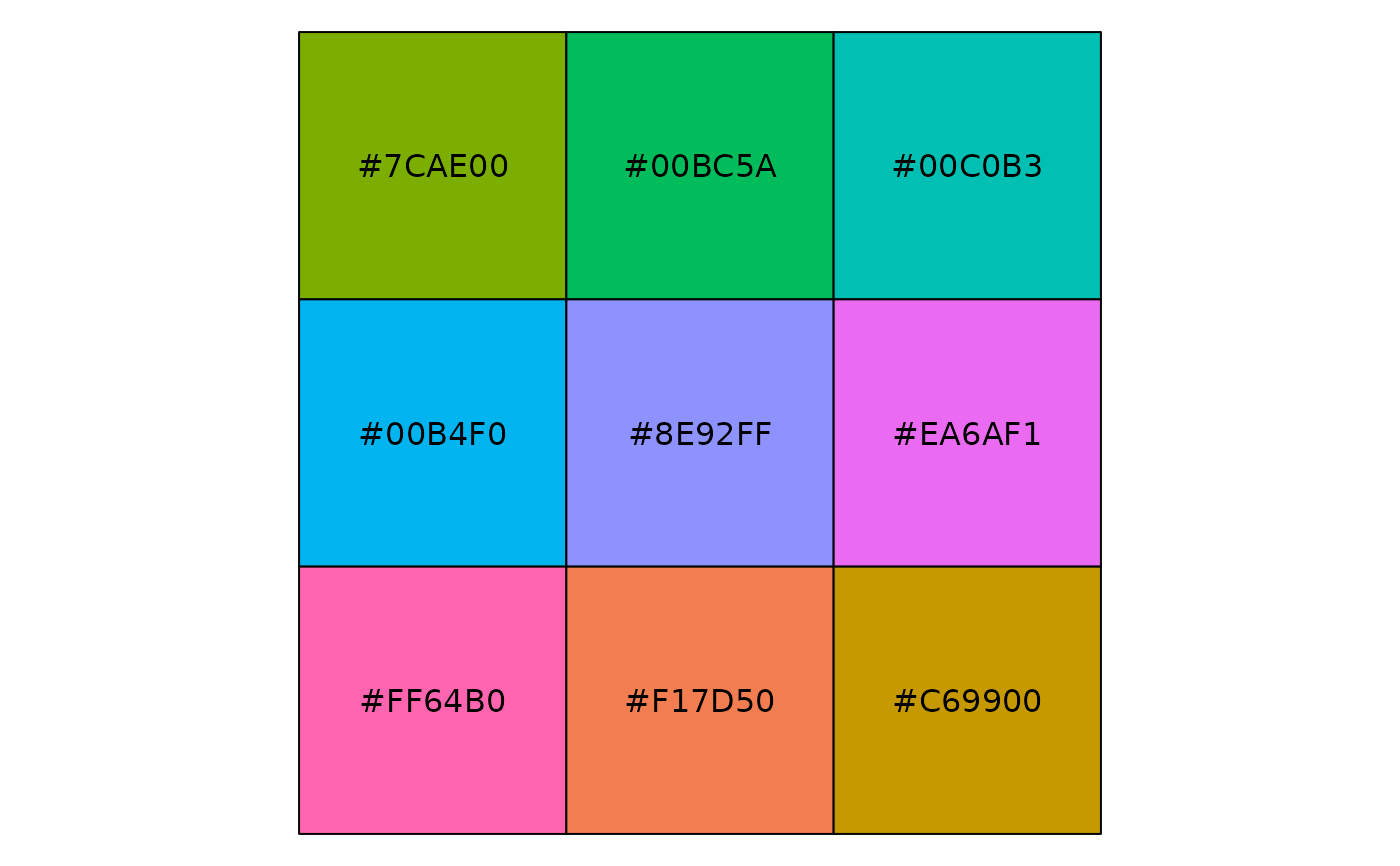show_col(hue_pal(h = c(90, 180))(9))show_col(hue_pal(h = c(180, 270))(9))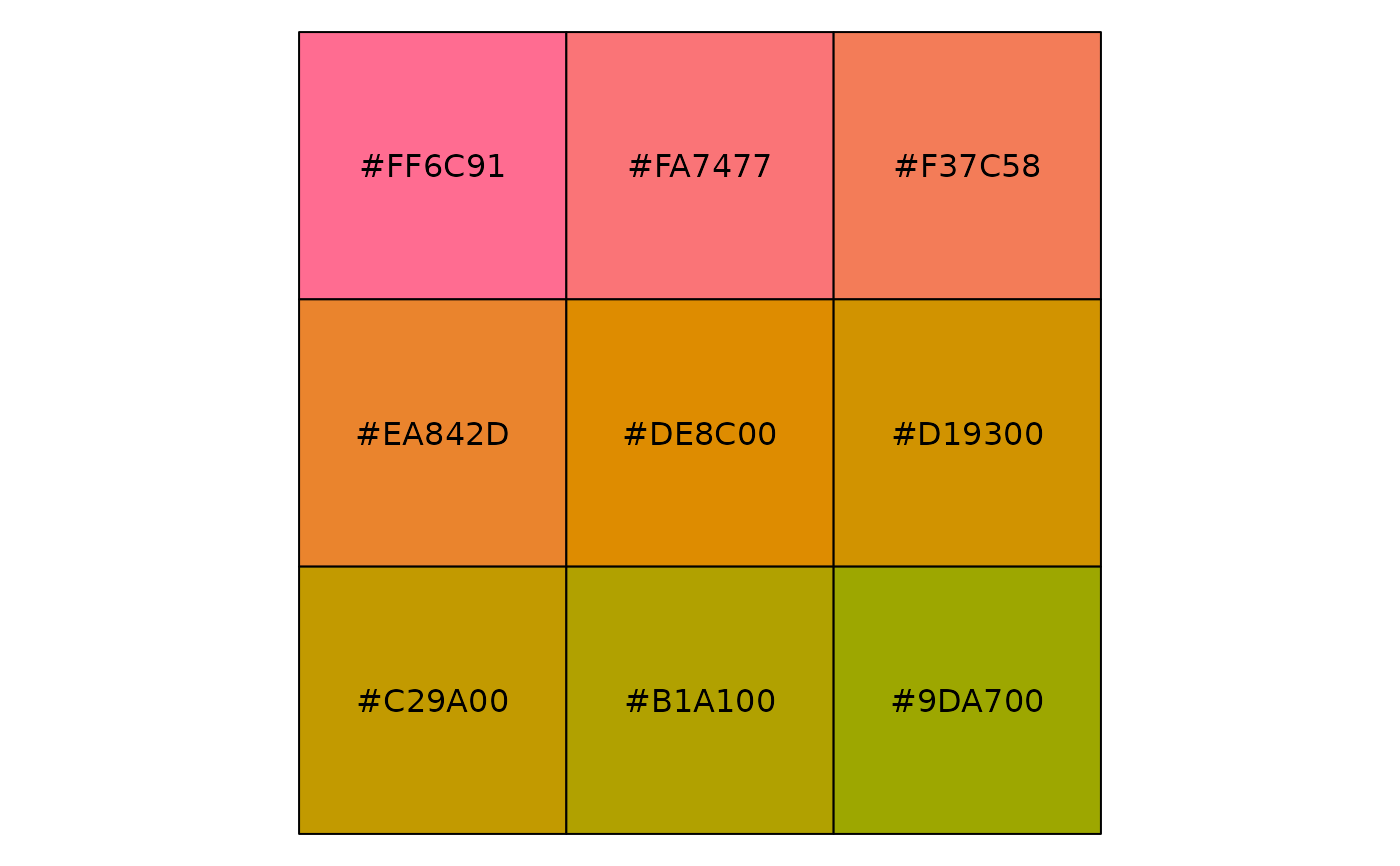show_col(hue_pal(h = c(270, 360))(9))